# Home | Mathematics | Pre-Algebra

## Pre-AlgebraBasic Operations With NegativesSimplifying ExpressionsSolving BinomialsUsing DivisionUsing MultiplicationMultiplication & DivisionVariable on One SideVariable on Both SidesWith Parentheses and MultiplicationEliminating Denominators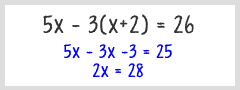Using Distributive PropertyMixed Problem TypesPolynomialsRoots of a polynomial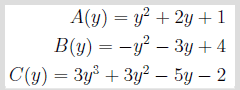Operations with PolynomialsSystems with 2 variables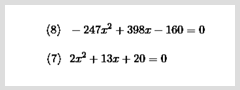Quadratic equation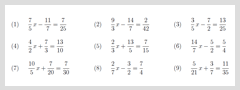Equations with fractions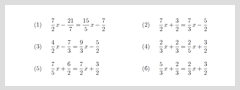Equations with fraction 2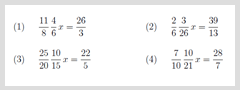Equations with fractions 3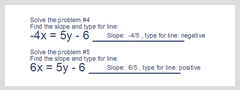Slopes and Types for linesTrinomialBinomial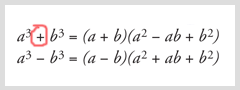Sum of Two Cubes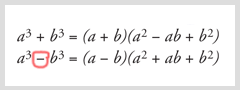Difference of Two Cubes